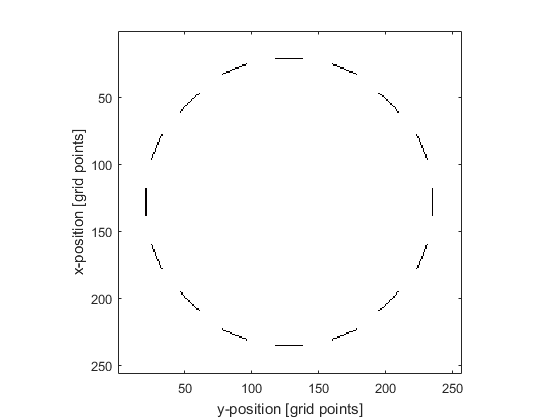makeMultiArc

Create a binary map of multiple arcs within a 2D grid.

Syntax

[arcs, arcs_labelled] = makeMultiArc(grid_size, arc_pos, radius, diameter, focus_pos)
[arcs, arcs_labelled] = makeMultiArc(grid_size, arc_pos, radius, diameter, focus_pos, ...)

Description

makeMultiArc creates a binary map of multiple arcs within a two-dimensional grid using makeArc. The position of the arcs is denoted by 1's in the matrix with 0's elsewhere. A labelled matrix can also be returned, where the position of the first arc is denoted by 1's, the position of the second arc by 2's, and so on.

Examples

% define grid parameters
x_size              = 300e-3;
Nx                  = 256;
dx                  = x_size / Nx;
grid_size           = [Nx, Nx];

% create a Cartesian circle with the x, y positions of the arcs
num_arcs            = 16;
arc_pos             = makeCartCircle(circle_radius, num_arcs, [1, 1] * x_size / 2).';

% convert the Cartesian arc positions to grid points
arc_pos             = round(arc_pos/dx);

% define element parameters
radius              = round(x_size / (2 * dx));
diameter            = 21;
focus_pos           = [1, 1] * Nx/2;

% create arcs
makeMultiArc(grid_size, arc_pos, radius, diameter, focus_pos, 'Plot', true);Inputs

 grid_size size of the 2D grid given as a two element vector [Nx, Ny] [grid points] arc_pos midpoint of each arc given as a matrix of dimensions N x 2, with each row specifying the midpoint of each arc as a two element vector [ax, ay] [grid points] radius radius of curvature of each arc given as either a single number (if the arcs have the same radius), or an N-element vector containing the radius for each arc [grid points] diameter diameter of each arc (length of straight line between the end points) given as either a single number (if the arcs have the same diameter), or an N-element vector containing the diameter for each arc [grid points] focus_pos any point on the beam axis of the arc given as either a two element vector (if the arcs have the same focus_pos), or as a matrix of dimensions N x 2, with each row specifying the focus_pos for each arc given as a two element vector [fx, fy] [grid points]

Optional Inputs

Optional 'string', value pairs that may be used to modify the default computational settings.

Input Valid Settings Default Description
'Plot' (Boolean scalar) false Boolean controlling whether the arc array is plotted using imagesc.

Outputs

 arc 2D binary map of an arc arcs_labelled 2D labelled matrix of arcs